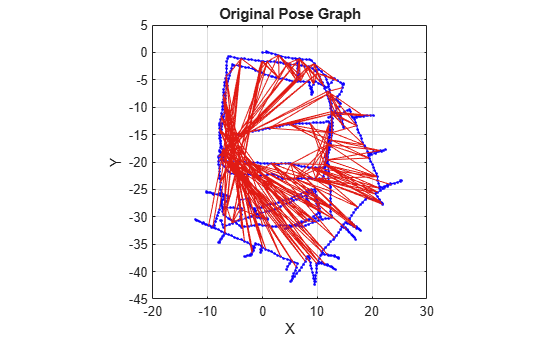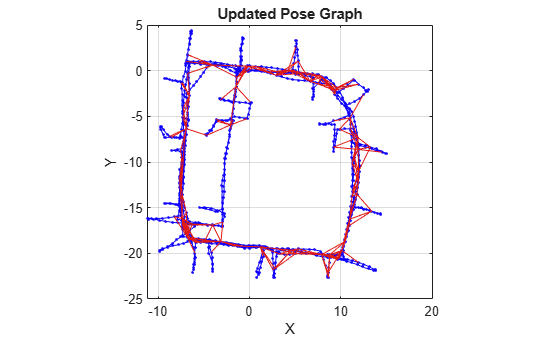# poseGraph

Create 2-D pose graph

## Description

A `poseGraph` object stores information for a 2-D pose graph representation. A pose graph contains `nodes` connected by `edges`, with edge constraints that define the relative pose between nodes and the uncertainty on that measurement. The `optimizePoseGraph` function modifies the nodes to account for the uncertainty and improve the overall graph.

For 3-D pose graphs, see `poseGraph3D`.

To construct a pose graph iteratively, use `addRelativePose` to add a node and connect it to an existing node with specified edge constraints. Specify the uncertainty of the measurement using an information matrix. Adding an edge between two existing nodes creates a loop closure in the graph.

`lidarSLAM` (lidar-based simultaneous localization and mapping) is built around the optimization of a 2-D pose graph.

## Creation

### Syntax

``poseGraph = poseGraph``
``poseGraph = poseGraph('MaxNumEdges',maxEdges,'MaxNumNodes',maxNodes)``

### Description

example

``` `poseGraph = poseGraph` creates a 2-D pose graph object. Add poses using `addRelativePose` to construct a pose graph iteratively.```
``` `poseGraph = poseGraph('MaxNumEdges',maxEdges,'MaxNumNodes',maxNodes)` specifies an upper bound on the number of edges and nodes allowed in the pose graph when generating code. This limit is only required when generating code.```

## Properties

expand all

Number of nodes in pose graph, specified as a positive integer. Each node represents a pose in the pose graph as an `[x y theta]` vector with an xy-position and orientation angle, `theta`. To specify relative poses between nodes, use `addRelativePose`. To get a list of all nodes, use `nodes`.

Number of edges in pose graph, specified as a nonnegative integer. Each edge connects two nodes in the pose graph. Loop closure edges are included.

Number of loop closures in pose graph, specified as a nonnegative integer. To get the edge IDs of the loop closures, use the `LoopClosureEdgeIDs` property.

Loop closure edges IDs, specified as a vector of edge IDs.

## Object Functions

 `addRelativePose` Add relative pose to pose graph `edges` Edges in pose graph `edgeConstraints` Edge constraints in pose graph `edgeResidualErrors` Compute pose graph edge residual errors `findEdgeID` Find edge ID of edge `nodes` Poses of nodes in pose graph `optimizePoseGraph` Optimize nodes in pose graph `removeEdges` Remove loop closure edges from graph `show` Plot pose graph

## Examples

collapse all

Optimize a pose graph based on the nodes and edge constraints. The pose graph used in this example is from the Intel Research Lab Dataset and was generated from collecting wheel odometry and a laser range finder sensor information in an indoor lab.

Load the Intel data set that contains a 2-D pose graph. Inspect the `poseGraph` object to view the number of nodes and loop closures.

```load intel-2d-posegraph.mat pg disp(pg)```
``` poseGraph with properties: NumNodes: 1228 NumEdges: 1483 NumLoopClosureEdges: 256 LoopClosureEdgeIDs: [1x256 double] ```

Plot the pose graph with IDs off. Red lines indicate loop closures identified in the dataset.

```show(pg,'IDs','off'); title('Original Pose Graph')```Optimize the pose graph. Nodes are adjusted based on the edge constraints and loop closures. Plot the optimized pose graph to see the adjustment of the nodes with loop closures.

```updatedPG = optimizePoseGraph(pg); figure show(updatedPG,'IDs','off'); title('Updated Pose Graph')```Grisetti, G., R. Kummerle, C. Stachniss, and W. Burgard. "A Tutorial on Graph-Based SLAM." IEEE Intelligent Transportation Systems Magazine. Vol. 2, No. 4, 2010, pp. 31–43. doi:10.1109/mits.2010.939925.